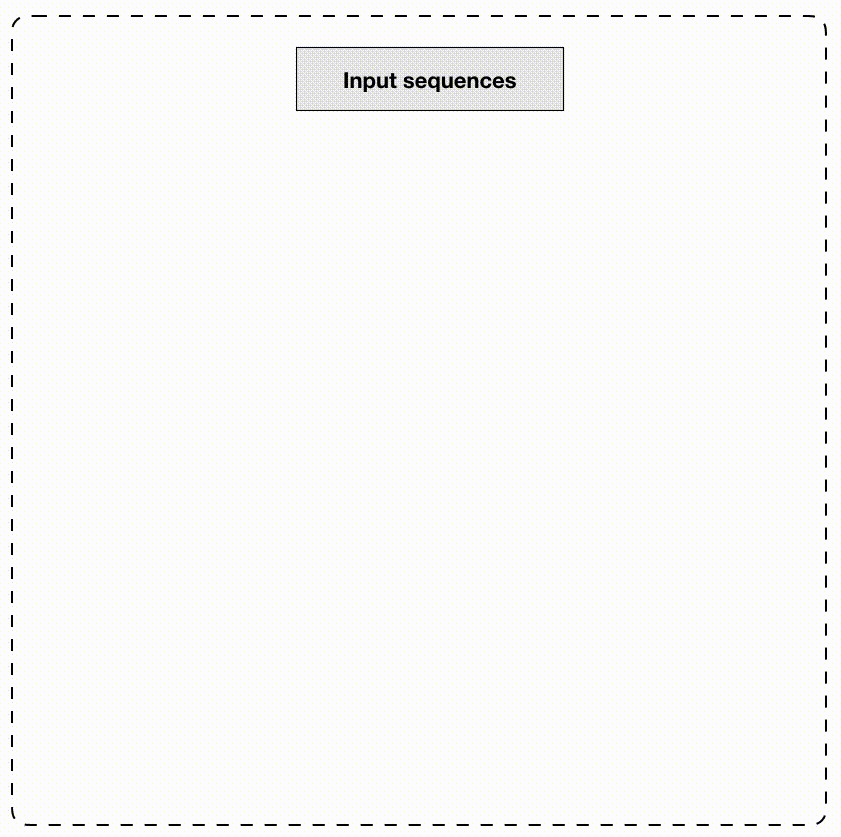seqArchR is an unsupervised, non-negative matrix factorization (NMF)-based algorithm for discovery of sequence architectures de novo. Below is a schematic of seqArchR’s algorithm.## Installation

### Python scikit-learn dependency

This package requires the Python module scikit-learn. Please see installation instructions here.

### To install this package, use

if (!requireNamespace("remotes", quietly = TRUE)) {
install.packages("remotes")
}

remotes::install_github("snikumbh/seqArchR", build_vignettes = FALSE)

### Usage

# load package
library(seqArchR)
library(Biostrings)

# Creation of one-hot encoded data matrix from FASTA file
inputFastaFilename <- system.file("extdata", "example_data.fa",
package = "seqArchR",
mustWork = TRUE)

# Specifying dinuc generates dinucleotide features
inputSeqsMat <- seqArchR::prepare_data_from_FASTA(inputFastaFilename,
sinuc_or_dinuc = "dinuc")

inputSeqsRaw <- seqArchR::prepare_data_from_FASTA(inputFastaFilename,
raw_seq = TRUE)

nSeqs <- length(inputSeqsRaw)
positions <- seq(1, Biostrings::width(inputSeqsRaw))

# Set seqArchR configuration
# Most arguments have default values
seqArchRconfig <- seqArchR::set_config(
parallelize = TRUE,
n_cores = 2,
n_runs = 100,
k_min = 1,
k_max = 20,
mod_sel_type = "stability",
bound = 10^-6,
chunk_size = 100,
result_aggl = "ward.D",
result_dist = "euclid",
flags = list(debug = FALSE, time = TRUE, verbose = TRUE,
plot = FALSE)
)

#
### Call/Run seqArchR
seqArchRresult <- seqArchR::seqArchR(config = seqArchRconfig,
seqs_ohe_mat = inputSeqsMat,
seqs_raw = inputSeqsRaw,
seqs_pos = positions,
total_itr = 2,
set_ocollation = c(TRUE, FALSE))

# Contact

Comments, suggestions, enquiries/requests are welcome! Feel free to email or create an new issue